# Heavy Metal Analogue

In order to explain the significance of the following story, some prequels are required.

Prequel #1: I’m one of those people who enjoys an addiction to collecting what other people call “junk” – things you find in flea markets, estate sales, and the like. Normally I only come home with old fountain pens that need to be restored, however, occasionally, I stumble across other things.

Prequel #2: Many people have vinyl records lying around, but not many people know how they’re made. The LP that you put on your turntable was pressed from a glob of molten polyvinyl-chloride (PVC), pressed between two circular metal plates called “stampers” that had ridges in them instead of grooves. Easy of those stampers was made by depositing layers of (probably) nickel on another plate called a “metal mother” which is essentially a metal version of your LP. That metal mother was made by putting layers on a “metal master” (also with ridges instead of grooves) which was probably a lamination of tin, silver, and nickel that was deposited in layers on an acetate lacquer disc, which is the original, cut on a lathe. (Yes, there are variations on this process, I know…) The thing to remember in this process is

• there are three “playable” versions of the disc in this manufacturing process: your LP, the metal mother, and the original acetate that was cut on the lathe
• there are two other non-playable versions that are the mirror images of the disc: the metal master and the stamper(s).

(If you’d like to watch this process, check out this video.)

Prequel #3: One of my recurring tasks in my day-job at Bang & Olufsen is to do the final measurements and approvals for the Beogram 4000c turntables. These are individually restored by hand. It’s not a production-line – it really is a restoration process. Each turntable has different issues that need to be addressed and fixed. The measurements that I do include:

• verification of the gain and response of the two channels in the newly-built RIAA preamplifier
(this is done electrically, by connecting the output of my sound card into the input of the RIAA instead of using a signal from the pickup)
• checking the sensitivity and response of the two channels from vinyl to output
• checking the wow and flutter of the drive mechanism
• checking the channel crosstalk as well as the rumble

The last three of these are done by playing specific test tracks off an LP with signals on it, specifically designed for this purpose. There are sine wave sweeps, sine waves at different signal levels, a long-term sine wave at a high-ish frequency (for W&F measurements), and tracks with silence. (In addition, each turntable is actually tested twice for Wow and Flutter, since I test the platter and bearing before it’s assembled in the turntable itself…)

Prequel #4: Once-upon-a-time, Bang & Olufsen made their own pickup cartridges (actually, it goes back to steel needles). Initially the SP series, and then the MMC series of cartridges. Those were made in the same building that I work in every day – about 50 m from where I’m sitting right now. B&O doesn’t make the cartridges any more – but back when they did, each one was tested using a special LP with those same test tracks that I mentioned above. In fact, the album that they used once-upon-a-time is the same album that I use today for testing the Beogram 4000c. The analysis equipment has changed (I wrote my own Matlab code to do this rather than to dust off the old B&K measurement gear and the B&O Wow and Flutter meter…)

If you’ve read those four pieces of information, you’ll understand why I was recently excited to stumble across a stamper of the Bang & Olufsen test LP, with a date on the sleeve reading 21 March, 1974. It’s funny that, although the sleeve only says that it’s a Bang & Olufsen disc, I recognise it because of the pattern in the grooves (which should give you an indication of how many times I’ve tested the turntables) – even if they’re the mirror image of the vinyl disc.

Below, you can see my latest treasure, pictured with an example of the B&O test disc that I use. It hasn’t “come home” – but at least it’s moved in next-door.

• No, the test disc is no longer available – it never was outside of the B&O production area. However, if you can find a copy of the Brüel and Kjær QR 2010 disc, it’s exactly the same. I suspect that the two companies got together to produce the test disc in the 70s. However, there were also some publicly-available discs by B&O that included some test tones. These weren’t as comprehensive as the “real” test discs like the ones accompanying the DIN standards, or the ones from CBS and JVC.
• No, the metal master is no longer in good enough shape to use to make a new set of metal mothers and stampers. Too bad… :-(

P.P.S. If you’re interested in the details of how the tests are done on the Beogram 4000c turntables, I’ve explained it in the Technical Sound Guide, which can be downloaded using the link at the bottom of this page. That document also has a comprehensive reading list if you’re REALLY interested or REALLY having trouble sleeping.

# Fixed point vs. Floating Point

When an analogue audio signal is converted to a digital representation, the value of the level for each sample is rounded to the nearest quantisation step (because a digital audio system does not have an infinite resolution). I’ve talked about this in detail in a past posting.

When a sample value in a digital audio stream is stored or transmitted inside a piece of audio equipment or software, one of the choices the engineer can make is whether the value should be represented using a fixed point or a floating point system. These are related, but fundamentally different, and they have some effects on the audio signal that may be audible if you’re not careful…

Let’s lay down some basic points to start. We’ll say the following:

• Audio is a kind of AC signal that has a level that can vary between two values.
• For now, we’ll say that the limits on the range of values is -1 and +1, and it can be anything in between.
• We’re going to divide up that range into some finite number of steps and round the actual signal value to the closest usable value. (I’ll assume for this posting that you already understand that dither is your friend.)
• The value will be stored as a binary number somehow

The question that we’ll look at here is exactly how that binary value represents the number, and a little of what that means to the audio signal.

## Fixed Point Representation

The simplest way to represent the value is to divide the total range from the minimum to the maximum number into an equal number of steps, and round the signal’s value to the closest step. This is a really generalised description of a “fixed point” system.

For example, if we have a 3-bit number to play with, we’ll take the first bit and use that one to represent the + or – portion of the value (where 0 means “+” and 1 means “-“). For values from 0 up to (just under) the positive maximum, the other 2 bits are used to just count the steps, from 000 up to 011. The negative values start at the bottom and work their way up to 1 step below 0, from 100 to 111. This can be seen in Figure 1.Figure 1: A simplified representation of the use of quantisation steps in a 3-bit fixed point system.

If you look carefully at Figure 1, you’ll see that there is one extra negative step, since one of the positive steps is used to represent the value 0 in the middle. This means that, if the signal is symmetrical, then we will wind up using all of the possible quantisation values except for the bottom one (just like I’ve shown in the plot), however, for the rest of this discussion, we’ll be working with numbers that are so big that this one step doesn’t really matter, so I won’t mention it again.

If we are using a 3-bit number to represent the value, then we have a total number of 23 quantisation steps: 8 of them. Each time we add one more bit, we double the number of steps. So, for a 16-bit sample, we have 216, or 65,536 possible quantisation values. For a 24-bit sample, we have 224, or 16,777,216 steps.

By increasing the number of bits in the number, we don’t change the level (it still has a range of -1 to +1), we’re just increasing the resolution that we have to make the measurement. The higher the resolution, the lower the error, and so the lower the level of distortion (if we don’t dither) or noise (if we do) relative to the signal.

If you have a fixed-point system, and you want to calculate the difference in level between the maximum signal level and the noise floor, then you can use a somewhat simplified equation, shown below:

Dynamic Range In dB ≈ 6 * nBits – 3

As I said, this is simplified due to some rounding to keep the numbers nice, but the general idea is that you have a doubling of dynamic range for every extra bit (therefore 6 dB per bit) and you lose 3 dB for the (TPDF) dither (but that’s better than not having the dither and having distortion instead). If you wanted to do it properly, then you can use this math instead:

Dynamic Range In dB ≈ 20*log10(2nBits) – 20*log10(sqrt(2))

So, if you have a 16-bit fixed point system, you have about 93 dB of range from the loudest signal to the noise floor. If you have a 24-bit system, it’s about 141 dB.

Remember that the noise floor is constant (I’m assuming it’s dithered), so as the signal level drops below maximum the current signal to noise ratio will drop by the same amount. Therefore, if your signal is 12 dB below maximum (or -12 dB FS, which means “12 decibels below Full Scale”), then the SNR in a 16-bit system is 93 – 12 = 81 dB.

If that last paragraph didn’t make complete sense, go back and read it again, because it’ll come back later…

Fixed point is a good system for conversion of an audio signal from and to analogue, but if you’re doing some really serious processing, it might not work out so well. This is due to two primary reasons:

• If your signal is going to outside the range, it will clip at the maximum positive or the minimum negative value because fixed point is not designed to exceed its range.
• If the signal is going to be reduced to a very low level somewhere in your proceeding (say, inside a biquad, for example) then you might need a LOT of bits to keep the noise floor low enough when the signal level is brought back up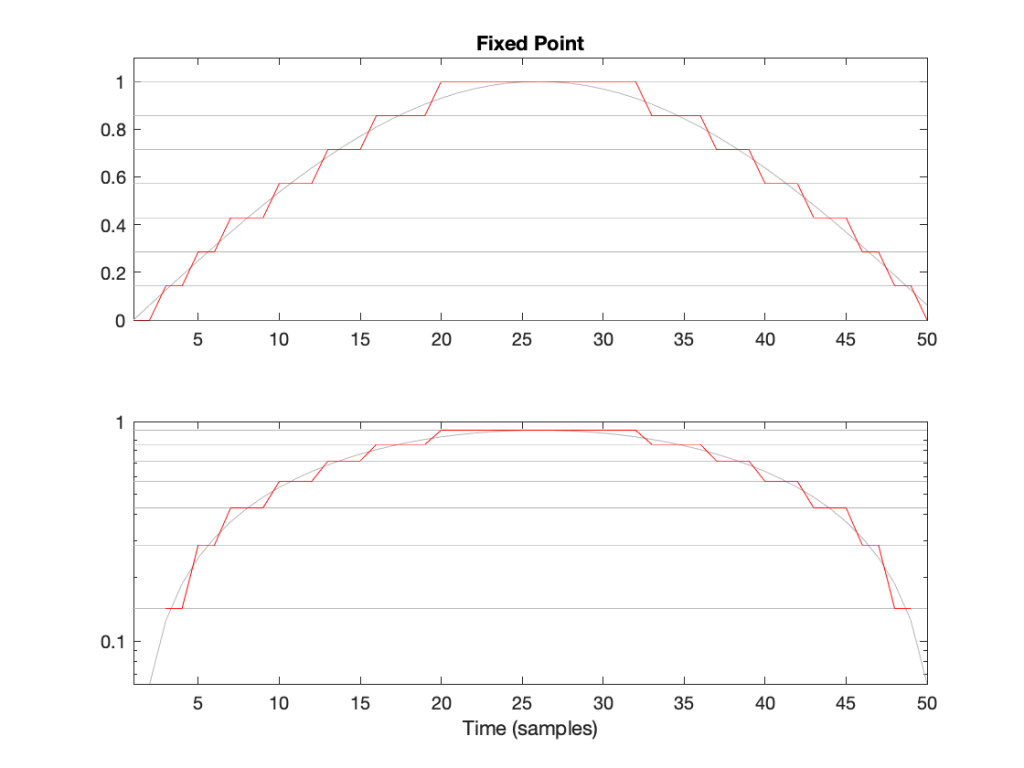Figure 2: The first half of a sine wave (in grey) quantised (without dither) in a simplified 4-bit fixed point system. (I’ve actually cheated a bit and just made 8 equally-spaced steps from 0 to 1 unlike the version shown in Figure 1.) The two plots show identical data, but the bottom plot has a logarithmically-scaled Y-axis.

As can be seen in Figure 2, the equally-spaced steps in a fixed point world mean that the quantisation error is always between -0.5 and 0.5 of a step (a “Least Significant Bit” or LSB), regardless of the level of the signal.

## Floating Point Representation

There is another way to use the bits to represent the signal value. This is to divide the binary “word” into two parts and to do a little math involving some subtraction, multiplication, and an exponent to arrive at the value. Just like in the Fixed Point case, we’ll reserve one bit for the +/- indicator.

Let’s say that we have a 32-bit value to work with. We’ll divide this up into the following:

• 23 bits for the fraction or mantissa, which we’ll abbreviate f
• 8 bits for the exponent, abbreviated e
• 1 bit for the +/- sign (just like in Fixed Point)

We’ll then do the following math:

Sample Value = ± (1 – f) * 2e

We need to know a little extra information:

• because we’re using 23 bits for f, then it can range from 0 to 223-1. In other words, stated mathematically:
0 ≤ 223*f < 223
• because we’re using 8 bits for e, then it has a total range of 28 possible values. In other words it has a range from just over -27 to just under 27. In other words, stated mathematically:
-126 ≤ e ≤ 127
(Note that a couple of possible values are reserved for special purposes, but we won’t talk about those)

This is all a little complicated, but there is a “punch line” to which I’m headed:

Unlike Fixed Point representation, the divisions of the values – the number of steps, and therefore the step sizes – are not the same across the entire scale of possible values. It’s divided into sections, where each section has quantisation steps of equal size, but that step size is dependent on what the value is. In other words the step size changes with the value, but on a coarser scale.

That step size can be calculated as follows:

From 2e to 2e+1, the steps all have an equal size of 2e-fBits where fBits is the number of bits used to express f (in the case of a 32-bit floating point word, fBits = 23 bits). In other words, we have 2fBits equally-spaced steps in that range.

Therefore, each time the signal value moves from just below 0.5 to just above (for example) then the resolution changes, and the higher the value, the lower the resolution. This is is how Floating Point representation behaves.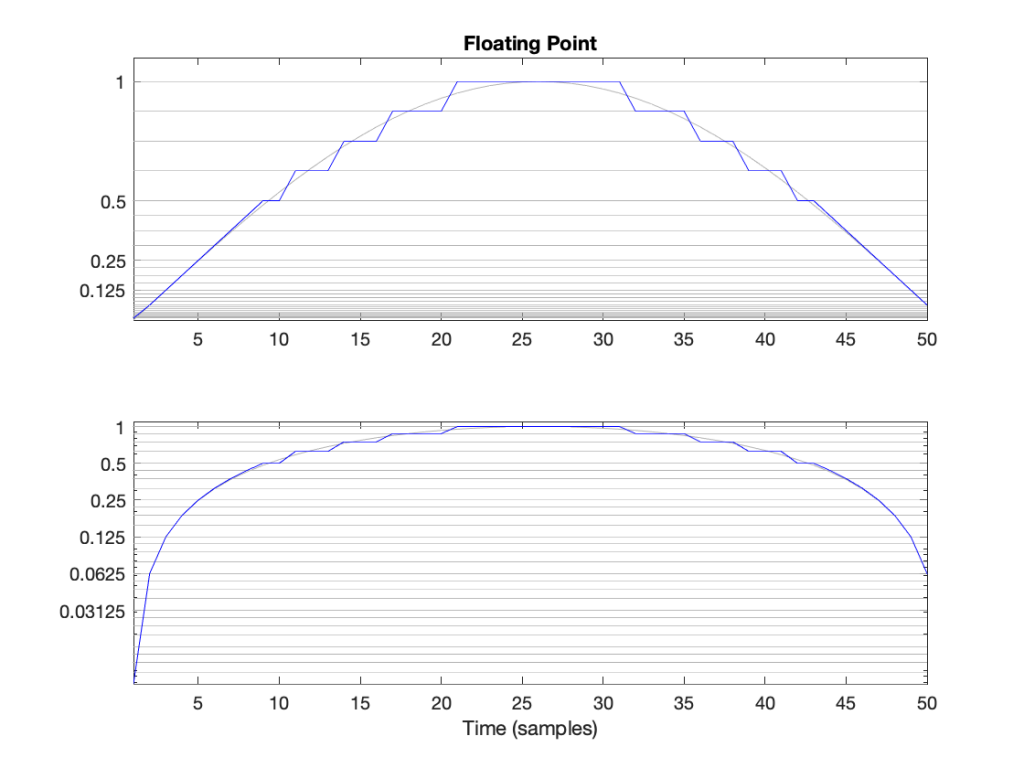Figure 3: The first half of a sine wave (in grey) quantised (without dither) in a simplified floating point system with 2 bits for the fraction. This means that there are 4 equally-spaced steps from (for example) 0.25 to 0.5 or 0.5 to 1. The two plots show identical data, but the top plot has a linearly-scaled Y-axis, whereas the bottom plot has a logarithmically-scaled Y-axis.

## Do I care?

Let’s find out.

In a 32-bit floating point world (therefore, one with a 23-bit fraction), if I have a signal that has level that has has a maximum positive value of 1 (or 20), then the resolution of the value (which defines the error, which defines the “distance” in dB to the noise floor) is 2-25 (or 1/33,554,432).* This means that the noise floor is about 150 dB below the signal (20 * log10(1 / 2-25). As the signal level drops to 0.5, the noise floor remains the same, so the signal drops by 6 dB, and the SNR reduces to 150 – 6 = 144 dB.

Then, when we drop just below 0.5, the resolution of the value suddenly changes to 2-26 (or 1/67,108,864) , which means that the noise floor is about 150 dB below the signal (20 * log10(0.5 / 2-26). As the signal drops to 0.25 (-6 dB relative to 0.5), the noise floor remains the same, so the signal drops by 6 dB, and the SNR reduces to 150 – 6 = 144 dB.

Then, when we drop just below 0.25, the resolution of the value suddenly changes to 2-27 (or 1/134,217,728), which means that the noise floor is about 150 dB below the signal (20 * log10(0.25 / 2-27). As the signal drops to 0.25 (-6 dB relative to 0.5), the noise floor remains the same, so the signal drops by 6 dB, and the SNR reduces to 150 – 6 = 144 dB.

Hopefully, by now, you’re seeing a pattern here.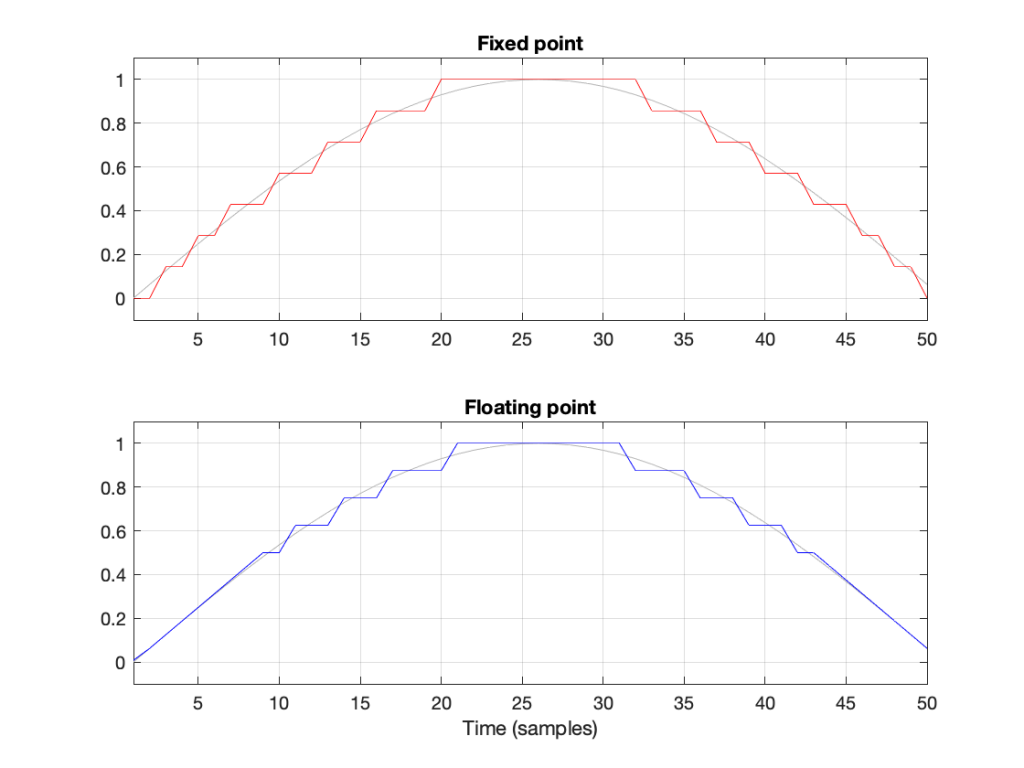Figure 4: Notice that the error of the floating point version is reduced when the signal level (in grey) approaches 0.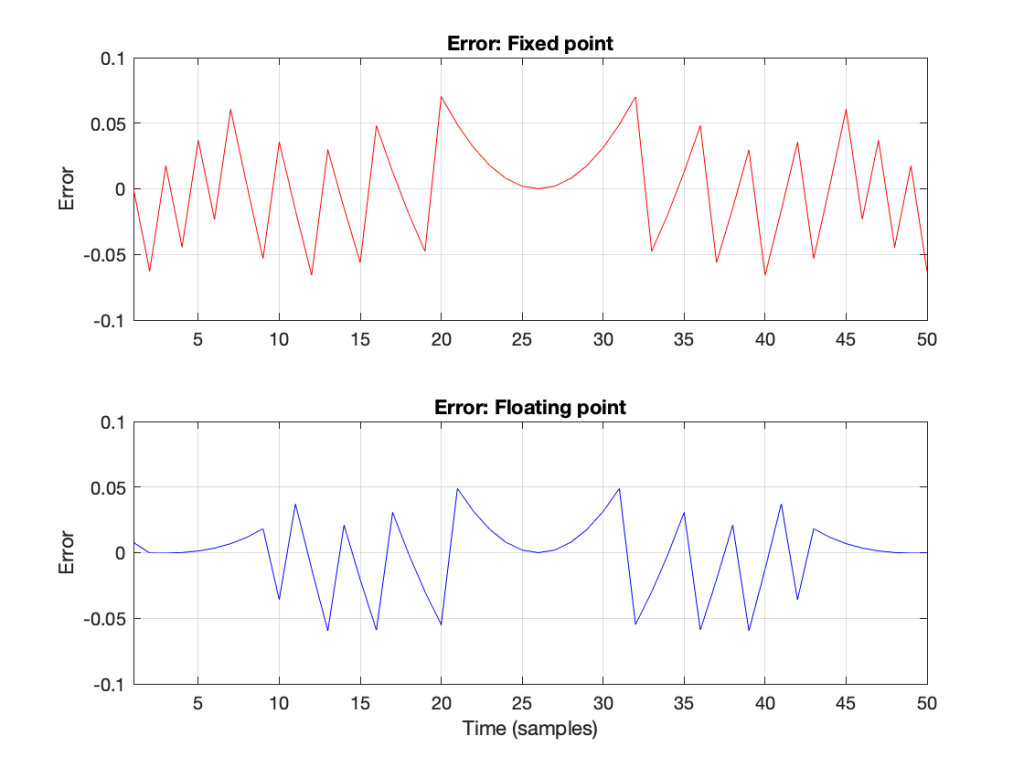Figure 6: The errors from the quantisation shown Figure 5. These are just the original signal subtracted from the quantised signals. Notice that, in Floating Point, the general level of the error is dependent on the level of the signal (it’s smaller on the left and right of the plot) whereas in Fixed Point, the overall level of the error is more constant.

The cool thing is that the pattern would have been the same if I had gone above 1 instead of below it. So, the two things to worry about in Fixed Point (inadequate resolution with (temporarily) low-level signals and clipping when the signal goes outside the range) are not problems in floating point.** And, if you have enough bits (32-bit floating point is the standard “single precision” resolution, but 64-bit “double precision” resolution is not uncommon).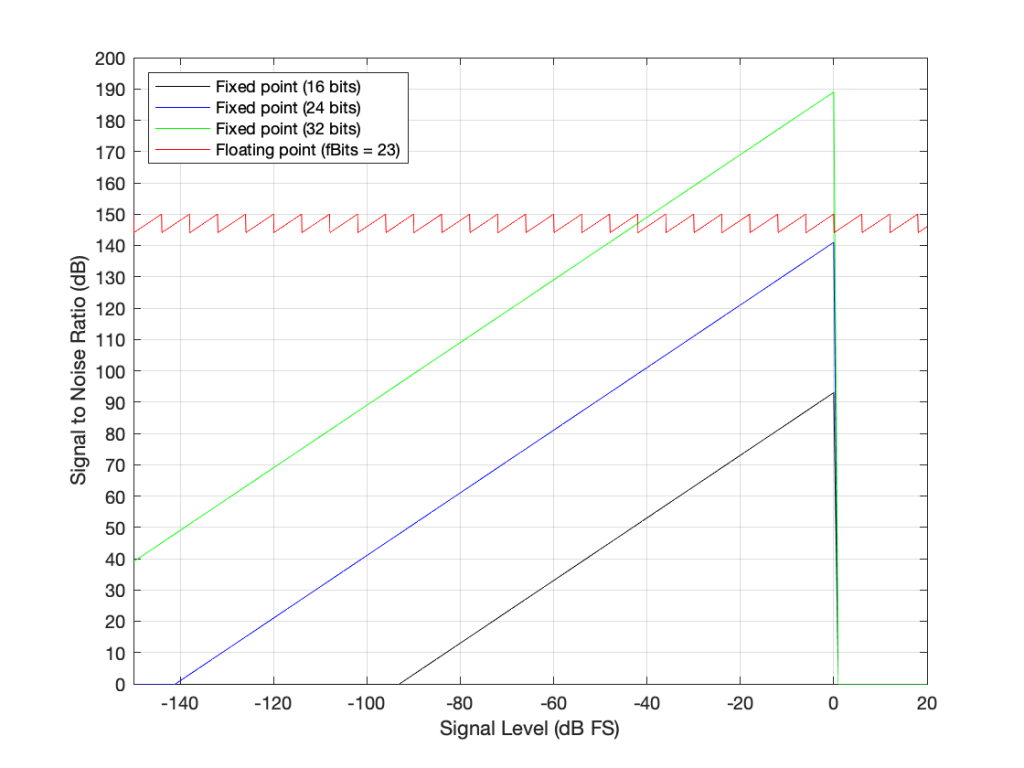Figure 7: The Signal to Distortion+Noise ratio of four different systems, as a function of the signal level in dB FS.**

This is why, in most modern audio systems, you have a fixed-point ADC and a DAC (an Analogue to Digital Converter and a Digital to Analogue converter) at the input and output of your system (because the signal range is reasonably well-defined, and the dynamic range is more than adequate if you do it right) but the processing on the inside is done in 32-bit or 64-bit floating point (or both, in some devices) so that the engineers have the resolution and the range to play with the signals before getting them ready for the output.***

There may be some argument made for a constant noise floor level in a fixed-point system (assuming it’s dithered) over a signal-modulated noise level in a floating-point world (assuming it’s not), however, there are two reasons why this is likely not a real-world issue. The first is that, even in a single-precision floating point system, the worst-case signal to noise ratio is about 144 dB, which is very good. The second is that smart people have already been thinking about dither for floating point systems. If this sounds interesting, you can start reading here

## * First small note for the attentive

You may have noticed what appears to be a mistake in my math in there. First I said:

From 2e to 2e+1, the steps all have an equal size of 2e-fBits where fBits is the number of bits used to express f (in our case, fBits = 23 bits). In other words, we have 2fBits equally-spaced steps in that range.

Then I did the math and said

In a 32-bit floating point world (therefore, one with a 23-bit fraction), if I have a signal that has level that has just come up to 1 (or 20), then the resolution of the value (which defines the error, which defines the “distance” in dB to the noise floor) is 2-25 (or 1/133,554,432).

Why did I say 2-25 when maybe I should have said 2-23 (because there are 23 bits in the fraction)? The reason is that the 223 quantisation levels are located between 1 down to 0.5. If I were to continue with the same spacing down to 0, then I would have twice as many quantisation levels, so there would be 224 instead. If I were to continue the spacing all the way down to -1, then there would be twice as many again, or 225.

In other words, a floating point signal ranging from a value of 2-1 to 20 (0.5 to 1) with some number of bits in the fraction that we’re calling fBits will have almost exactly the same signal to noise ratio as an non-dithered fixed point system that is scaled to range from -1 to 1 with fBits+2.

This would be the same from -20 to -2-1 (-1 to -0.5).

At any other signal value, the quantisation behaviours (and therefore the signal-to-noise ratios) of the two systems will be significantly different.

This is visible in Figure 6 where, when the signal is high (in the middle of the plots), the error level is approximately the same in the 4-bit fixed-point system and the floating point system with 2 bits for the fraction.

## ** Second small note for the attentive

You will notice that the black, blue, and green lines in Figure 7 have a sharp transition when the signal level hits 0 dB FS. This is because, in a fixed point system at signal levels below 0 dB FS, the signal to noise ratio is the difference in level between the dither’s noise floor and the signal. The dither level is constant, so as the signal level increases, it gets “further away” from the noise floor until you reach 0 dB FS (with a sine wave), as which point you reach the maximum possible SNR. However, once the signal goes beyond 0 dB FS (still assuming it’s a sine wave), then it starts to clip and distortion components are generated. It does not take much increase in level to drastically increase the level of the distortion relative to the level of the signal (since the signal level cannot increase – you’re just increasing distortion artefacts). Consequently, the signal to distortion+noise drops dramatically, because the distortion components increase in level dramatically.

This does not happen with the floating point system because, at 0 dB FS, you just change the exponent and keep going up with the signal level until you reach the maximum possible exponent value, which goes far beyond what I’ve plotted here.

## Third small note for the attentive

You may be looking at Figure 7 and wondering why the fixed point plots and the floating point plots don’t overlap anywhere. For example, look where the green line (32-bit fixed point) crosses the red line (32-bit floating point). Why don’t they overlap each other there for that little 6 dB-wide range on the X-axis?

The reason is that I’m modelling the fixed point SNRs with TPDF dither, which “costs” 3 dB, but I’m assuming that the floating point signal is not dithered (which would normally be the case). If I were pretending that fixed point didn’t include the dither, then the plots would, indeed, overlap each other for that narrow little window.

## ***One last comment

You may be saying to yourself “But this is nonsense! Why do I need 150 dB SNR when the signal level is lower than -100 dB FS?” The long answer is in this posting, but the short answer is that the signal can go VERY low and VERY high inside a filter (a biquad), so you need to worry about this if you’re doing any changes to the magnitude response of the signal, for example…

Floating Point Numbers posted by Cleve Moler at Mathworks

Floating Point Denormals, Insignificant But Controversial posted by Cleve Moler at Mathworks

# Quantisation of Poles in the Z-plane

Back in this posting, I talked about biquads and their use in digital signal processing for making linear filters (what most of us call “equalisers”). Part of that explanation showed that a biquad is formed of a feed-forward section and a feed-back section, and you could swap the order of these two and get the same results. In a “Direct Form 1” implementation, the feed-forward comes first, as shown in Figure 1.

in the “Direct Form 2” implementation, the feed-back comes first, as shown in Figure 2.

There are advantages and disadvantages to each of these implementations, depending on things like how the rest of your system is implemented, and what, exactly you’re expecting the biquad to do.

However, for this posting, we’re going to “zoom in” a bit to the feed-back portion of the above diagrams. This portion of the biquad provides the “poles” in the Z-plane, as I described in the “Intuitive Z-plane” series of postings last week.

If I separate the feed-back portion of the above figures, it would look like Figure 3:

The locations of the poles (and therefore the magnitude response) of this portion of the filter are dependent on the gains at the outputs of the two 1-sample delays. What happens when these gains do not have an infinite resolution (which they can’t, because everything in a digitally-represented world is quantised to a finite number of steps)?

Before we go any further with this, I have to put in a reminder that quantisation (or “rounding”) in a DSP world is done in binary – not decimal. So, the quantisation that I’m about to do isn’t the same as stopping a couple of digits after the decimal…

In a world with infinite resolution, I can set the values of those two gains to be anything I want, and therefore the poles in my system can be anywhere within the circle. (We’ll assume that we don’t want a pole on the circle because that would result in a gain of ∞ dB, which is very loud.) However, let’s say that we quantise the gain values to a 4-bit binary value, where the first bit is reserved for the +/- indicator. This means that we only have +/- 2^3 possibilities, or 8 values, one of which is +0, and another is -0 (which is the same thing…). So, in other words, we have 7 possible negative values, 7 possible positive values, and 0.

The end result of this is that the poles have a limited number of locations where they can be placed. For the 4-bit quantisation described above, the resulting locations look like Figure 4.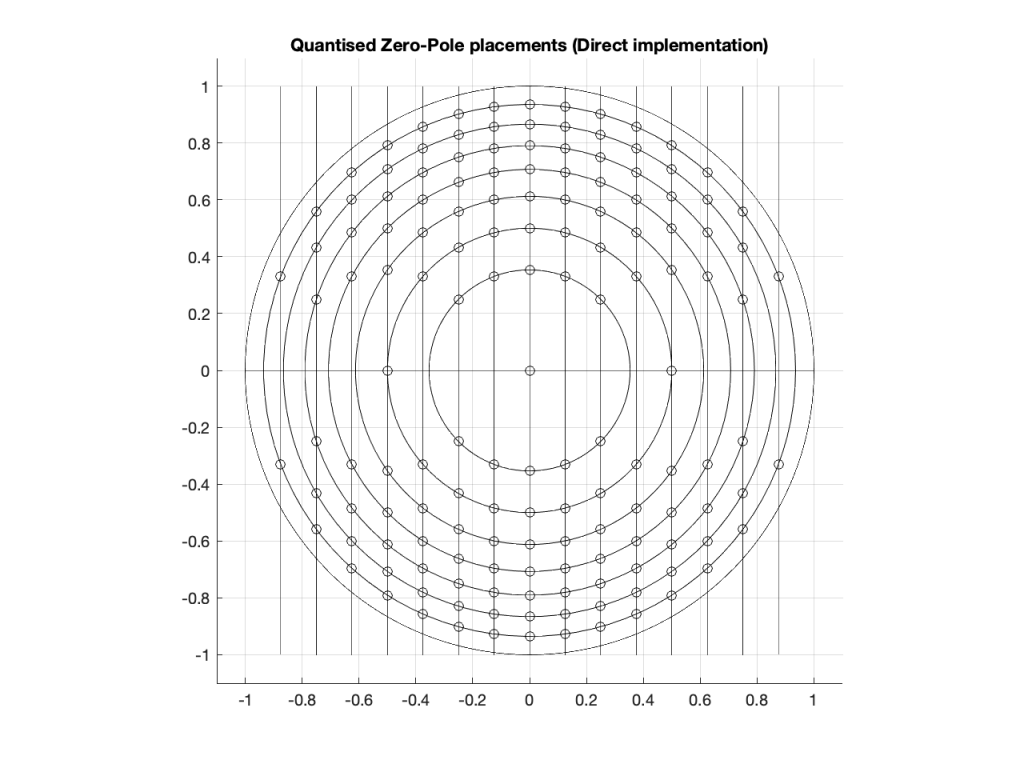Figure 4: The circles indicate the possible locations of the poles as a result of quantisation of the gain coefficients in a 4-bit Direct Form implementation.

Remember that we’re not talking about quantisation of the audio signal – it’s quantisation of the gain coefficients inside the biquad that will have an impact on the response of the filter.

Of course, it would be crazy to implement a biquad using only 4-bit quantisation for the gain coefficients. However, the point of this posting is not to show that biquads suck. It’s only to show one possibly important aspect of them if you’re a DSP engineer – but we’re getting there.

Just for fun, let’s increase the resolution of the system to 6 bits: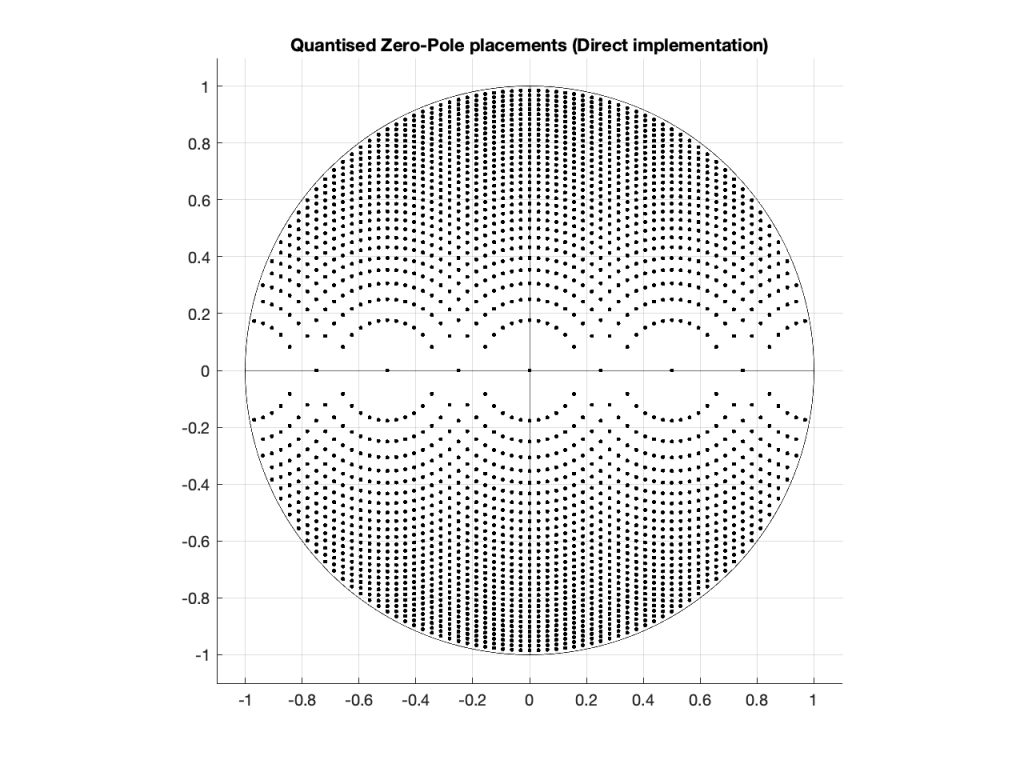Figure 5: The dots indicate the possible locations of the poles as a result of quantisation of the gain coefficients in a 6-bit Direct Form implementation.

… or to 8 bits: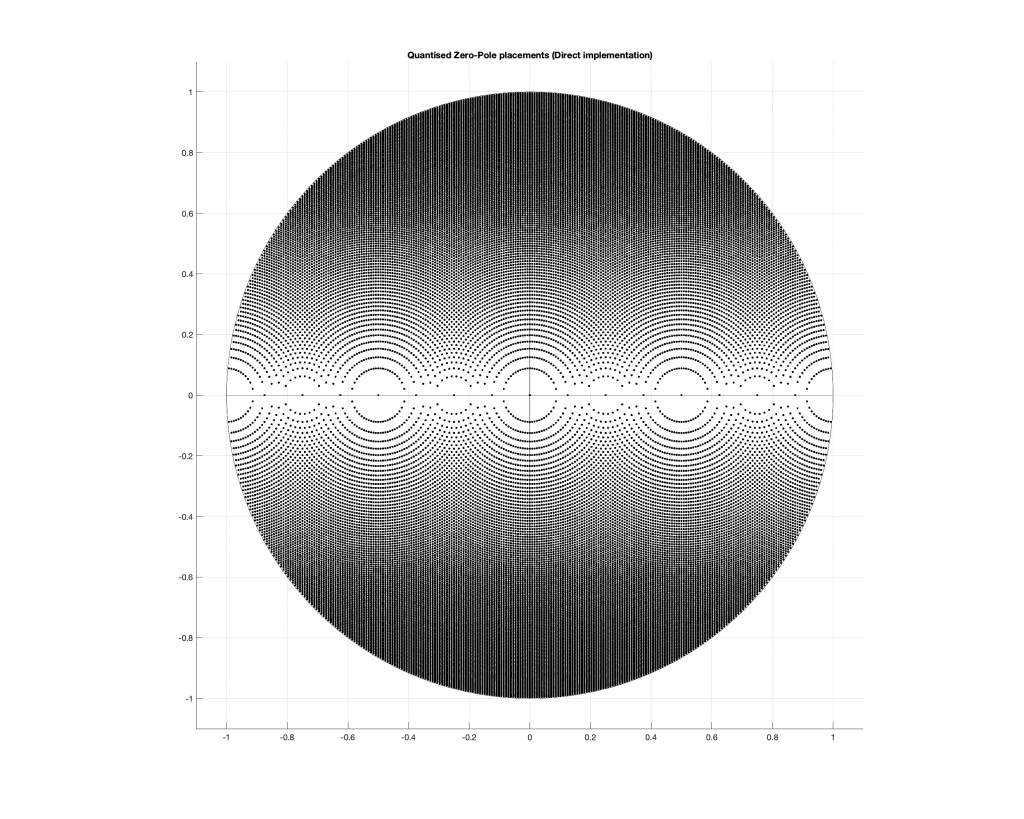Figure 6: The dots indicate the possible locations of the poles as a result of quantisation of the gain coefficients in a 4-bit Direct Form implementation.

Any higher than this and the pattern will just get so dense that it’ll turn black, so I’ll stop.

## So what?

At this point, you may be asking “so what?” The answer to this very important question lies on the far right side of all of those graphs. Notice that there aren’t any locations near the point on the graph where X=1 and Y=0. You may remember from Figure 7 in this posting that I did last week, that that’s the point on the Z-plane that corresponds to very low frequencies.

This means that, if you want to create a digital filter, and you need a pole near 0 Hz, you’re going to run into some trouble with a Direct Form implementation of the biquad. Yes, the higher the bit depth of the gain coefficients, the closer you can get, but this might not be the best way to do things.

There is another option for implementing your feed-back portion of the biquad. You can use a design called a Coupled Form instead, which is shown in Figure 7 (compare it to Figure 3).

Notice that you still have two 1-sample delays, however there are now 4 gains instead of 2. How can this be better? Well, in a system with infinite resolution on the gain coefficients, it’s not. Given the appropriate choice of gain values, this implementation will do exactly the same thing as the Direct Form implementation if your resolution is infinite.

However, if you have a limited resolution, then the available locations of the poles on the Z-plane are very different. Let’s use the 4-bit, 6-bit, and 8-bit quantisations of the gain values again: these are shown in Figures 8 to 10.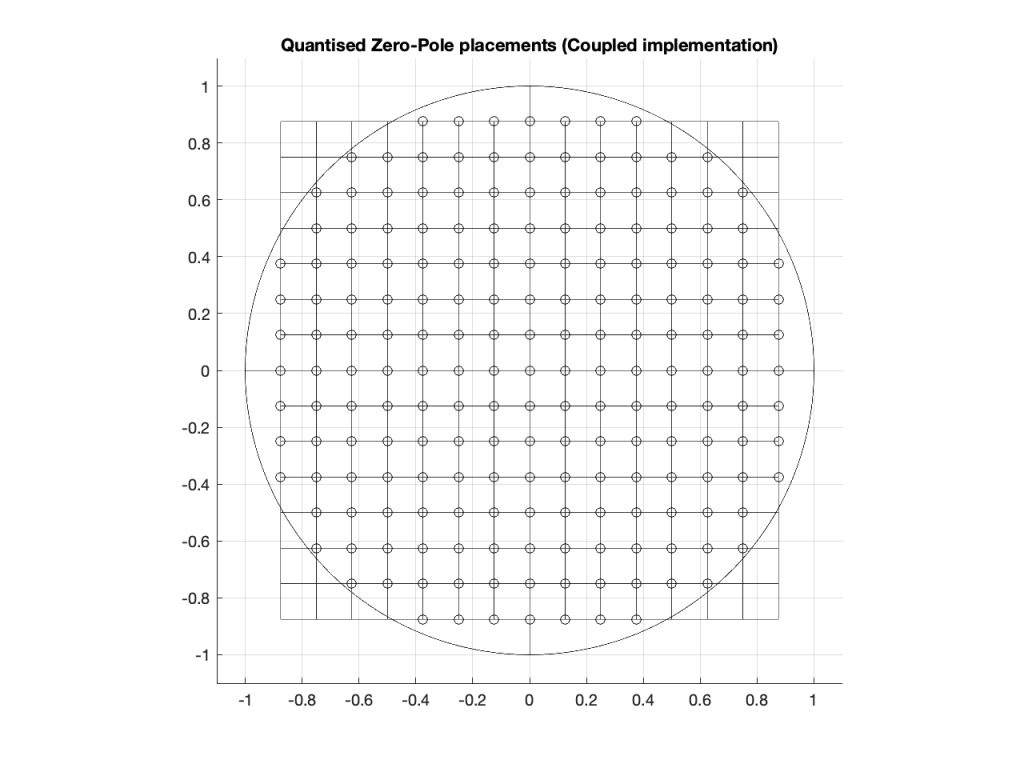Figure 8: The circles indicate the possible locations of the poles as a result of quantisation of the gain coefficients in a 4-bit Coupled Form implementation.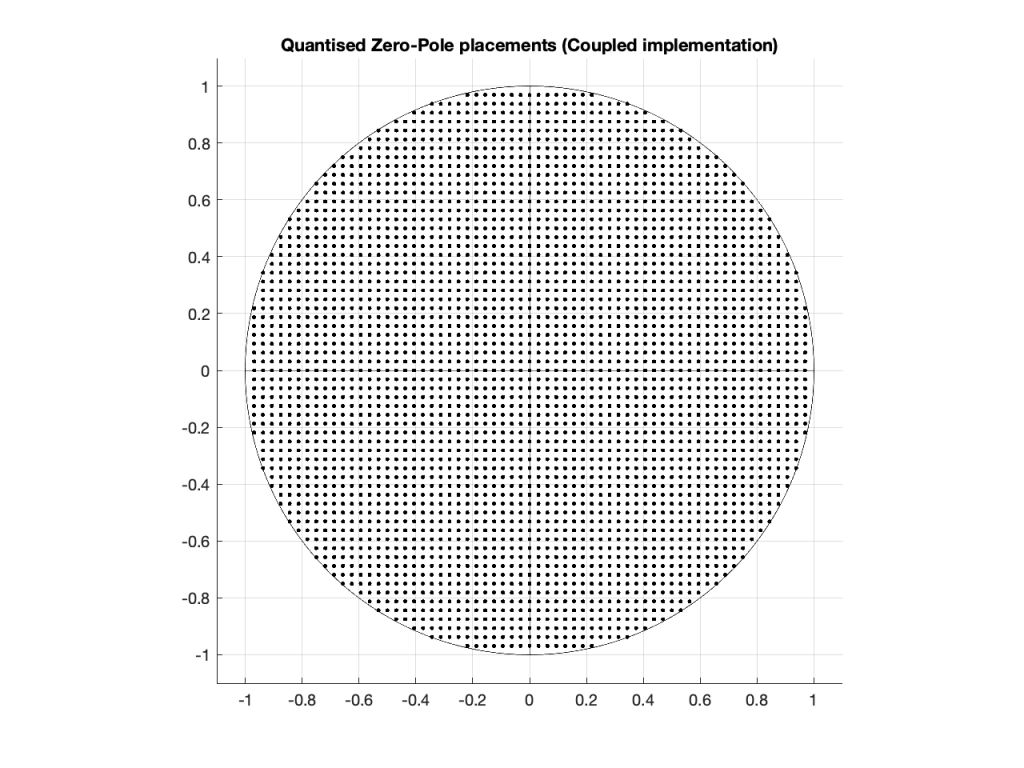Figure 9: The dots indicate the possible locations of the poles as a result of quantisation of the gain coefficients in a 6-bit Coupled Form implementation.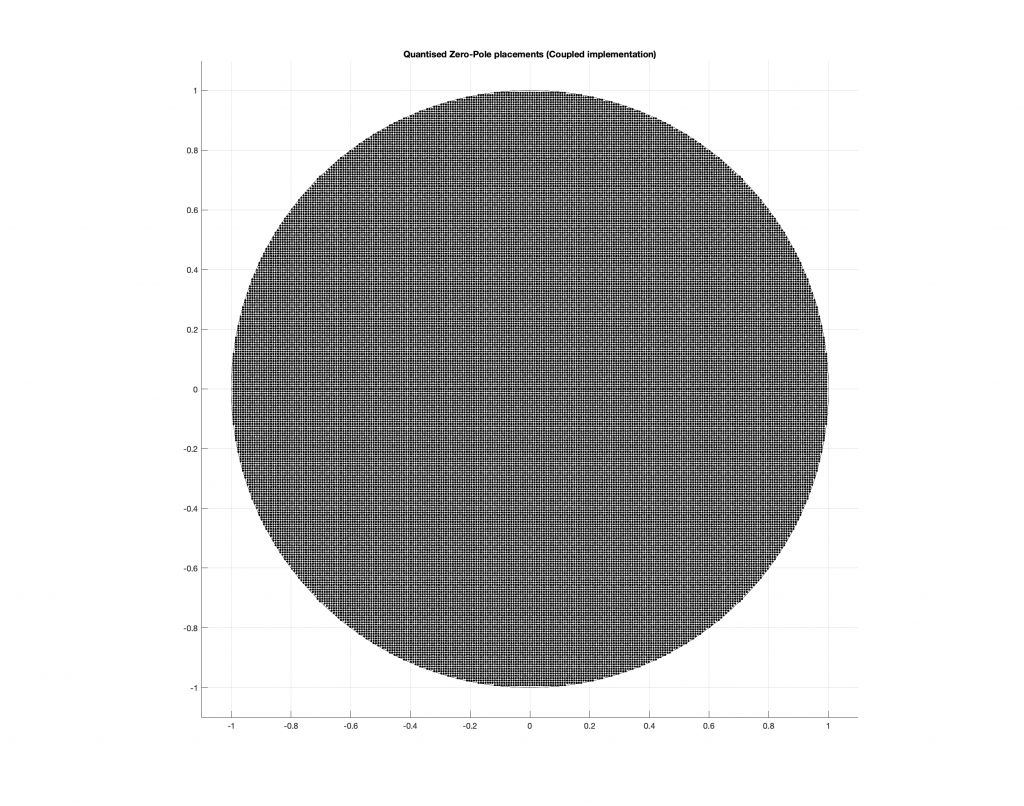Figure 10: The dots indicate the possible locations of the poles as a result of quantisation of the gain coefficients in a 8-bit Direct Form implementation.

As you can see in Figures 8 through 10, the Coupled Form implementation, given the same resolution on the gain coefficients, will give you much better placement opportunities for the poles in the low frequency region than the Direct Form implementation.

Of course, in all of these examples, I’m only showing up to an 8-bit word, and a typical DSP runs uses a lot more than 8 bits for the gain coefficients. So, it’s possible that, in the real world, the actual resolution is high enough that this is of no concern whatsoever.

However, if you’re building a very-low-frequency filter an if the magnitude response isn’t exactly what you’re looking for, this might help you get a little closer to your goal.

It’s important to point out here that the quantisation of the locations of the zeros is not the same as this. Someday, I’ll come back to this and plot the expected vs. actual magnitude responses of some biquads where I’ve quantised the zeros and poles, just to see how badly things go wrong when they do…

This posting is a simple summary of the discussion of a section called “Poles of Quantized Second-Order Sections” in “Discrete-Time Signal Processing” by Alan V. Oppenheim and Ronald W. Schafer.

The Coupled Form implementation was introduced by C.M. Rader and B. Gold in their paper called “Digital Filter Design Techniques in the Frequency Domain” from the Proceesings of the IEEE, Vol. 55, pp. 149 – 171 , from February, 1967.

# Intuitive Z-plane: Part 5 – Conclusion

If you’ve read through the first four parts of this series, then you’re already at a point where you can intuitively understand what’s going on. We just have a couple of details to take care of before finishing off.

Firstly, the plots showing the zeros and poles in the figures you’ve been looking at plots of the “Z-plane” or “Complex-plane“. As I said at the start, we’re only trying to get to an intuitive understanding of these plots – so I’m not going to get into complex numbers, or even much math (apart from what you’ll see below… which isn’t very complicated, and avoids complex numbers).

When I’m developing a new DSP algorithm, I use an application called Max from cycling74.com. Figure 1 shows a screenshot from Max, where I’m using an object to calculate the biquad coefficients to make a low pass filter, as you can see. I’ve then connected the output of that object (it looks like a magnitude response) to a Z-plan representation that shows me the same thing in a different way.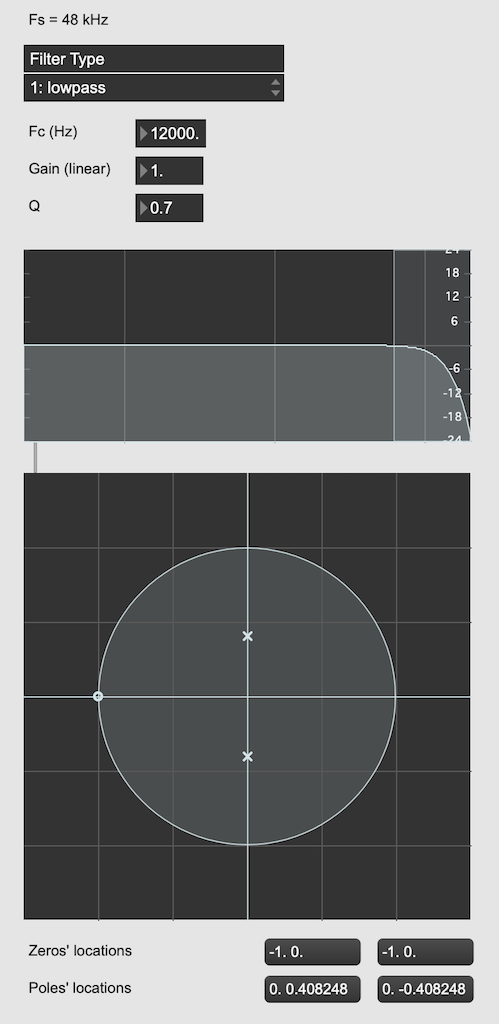Figure 1: The top plot shows the magnitude response of the filter. The bottom plot shows the Z-plane representation of the same filter.

You may notice that this plot has two poles, one at (0, 0.408) and the other at (0, -0.408). In fact there are two zeros there as well, but they’re situated in the same place, on “on top” of the other, at (-1, 0). This is always true for a biquad – there are always two zeros and two poles. Sometimes, they’re located in the same place, sometimes not, sometimes they’re placed symmetrically, sometimes not, depending on the filter, as we’ll see below.

Let’s look at that Z-plane representation in 3-dimensions: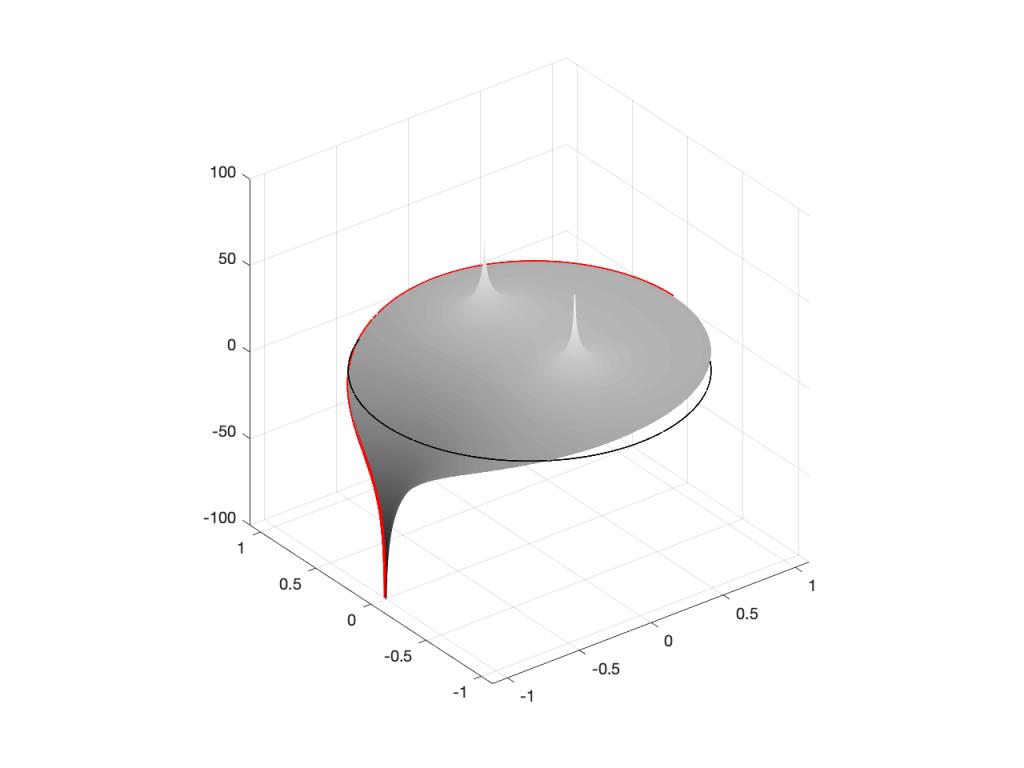Figure 2: A 3D view of the Z-plane representation of the filter shown in Figure 1.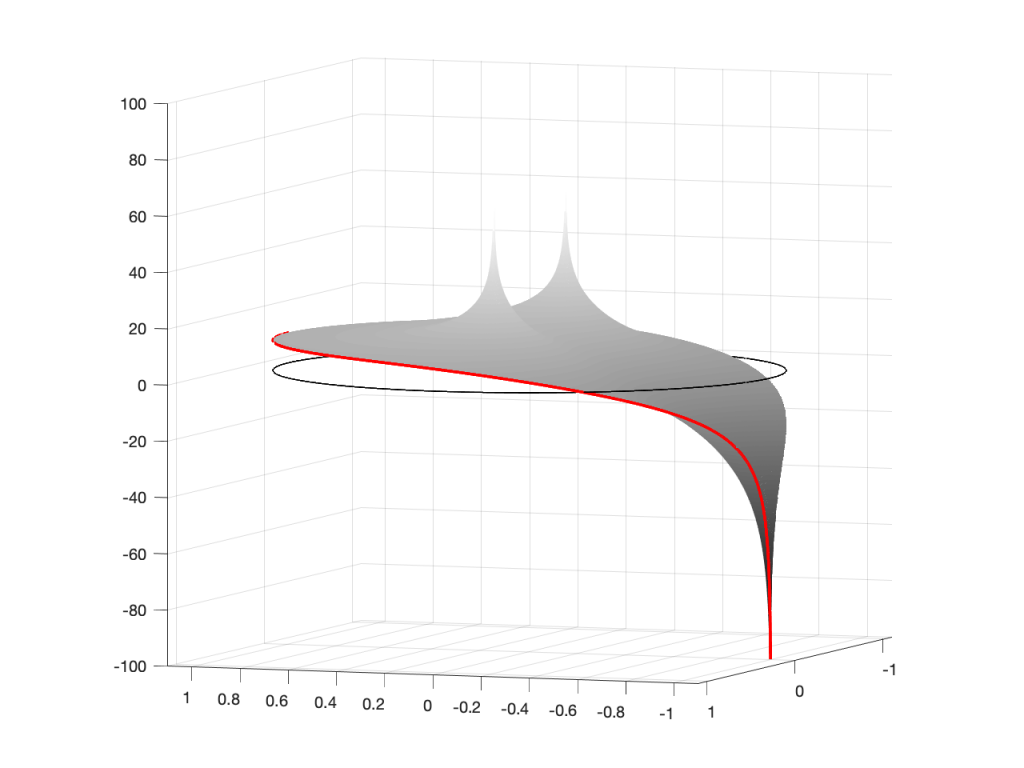Figure 3: The same plot as shown in Figure 2, rotated to show the back of the plot.

So, as you would now expect, the poles pull up the edge of the circle, and the zeros (both in the same place) pull down, giving the red line the height that it has.

Now, think back to this Figure from earlier in the series:

If you therefore look at Figure 3, which is like looking at Figure 4 from the top, you’ll notice that the height of the red line (the edge of the circle is high on the left (in the low frequencies) and drops as you go to the right (the high frequencies). This is the magnitude response that’s shown on the top of Figure 1. The only difference is that it’s on a linear scale instead of a logarithmic scale, so the shape looks a little weird.

Let’s do another one: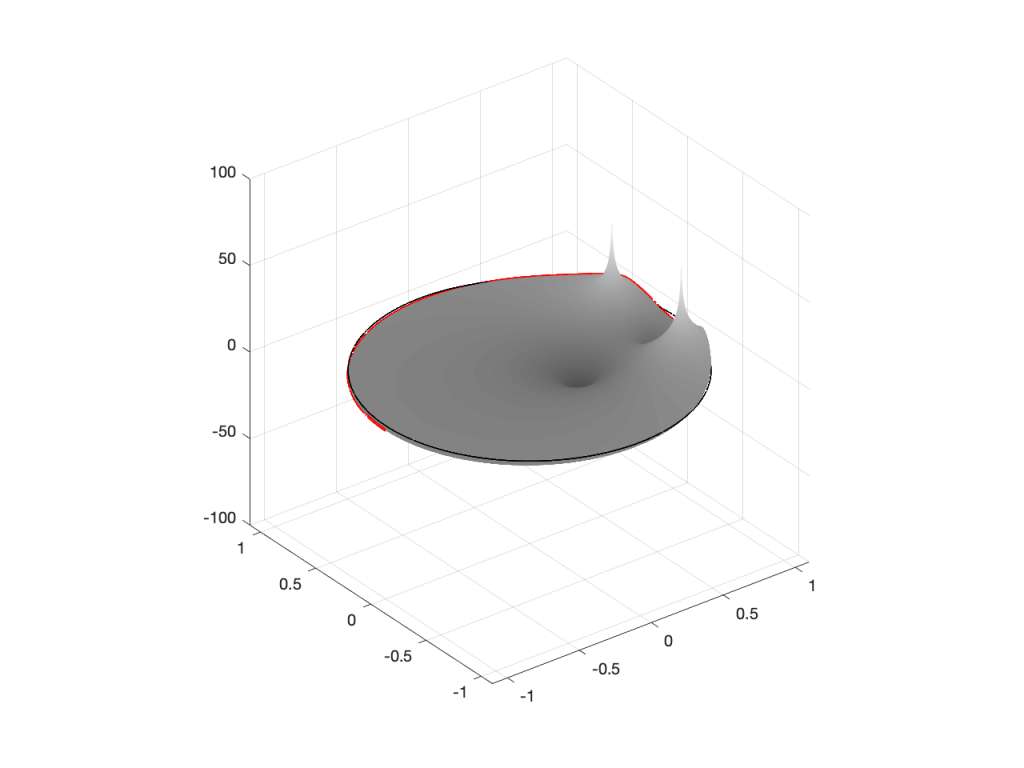Figure 6: A 3D view of the Z-plane representation of the filter shown in Figure 5.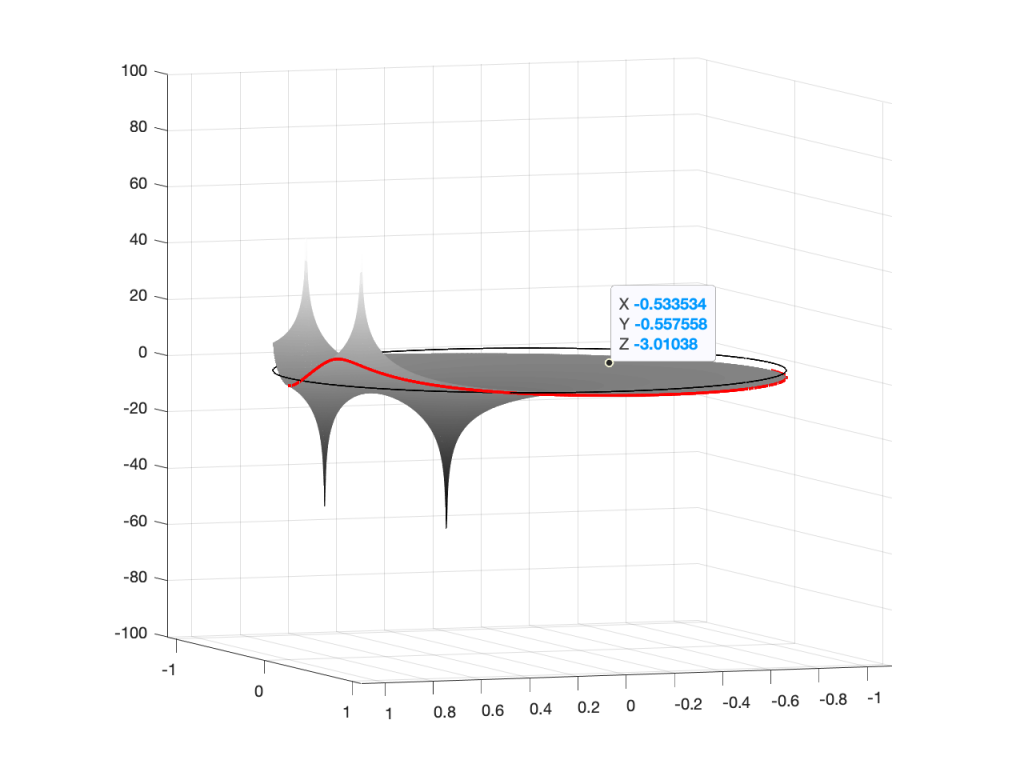Figure 7: The same plot as shown in Figure 6, rotated to show the back of the plot.

Hopefully, now you are able to look at a Z-plane representation of a filter and think about the effect of the poles and zeros on the edge of the circle, and therefore get a rough idea of the magnitude response of the filter…

If not, I apologize for wasting your time. On the other hand, if you’re in a life-threatening situation, this knowledge probably wouldn’t help you anyway… Very few people have gotten a critical injury in a biquad accident.

## How I did it

If you want to make these plots for yourself, the math is pretty simple.

Start by choosing the frequency, which will be a point on the circle. You then find the four distances from the zeros and poles to that point (I’ve indicated those distances in Figure 8 with the variables z1, z2, p1, and p2.) This can be done using the Pythagorean theorem.

To find the gain of the filter at the frequency, you divide the sum of the zeros’ distances by the sum of the poles’ distances. In other words:

(z1 + z2) / (p1 + p2)

That will give you the result as a linear value. If you then want to convert it to decibels, like I’ve done, you do a little extra math like this:

20 * log10 ( (z1 + z2) / (p1 + p2) )

That’s it! You just need to do repeat that math for each frequency that you’re interested in, and you’re done!

# Intuitive Z-plane: Part 4 – How, not what

I ended Part 3 by saying that DSP engineers think of the frequency scale as a circle rather than as a straight line. The questions are “Why do they think like this? What’s wrong with them?” Although I can’t answer the second question, the answer to the first question is fundamentally simple.

A DSP engineer is not really interested in what a filter does. She or he is interested in how it filters. A normal magnitude response plot shows us mortals the result of what’s happened to the audio after it’s gone through a filter (or a processor in general). Someone making that filter (or system) needs to know how it’s working instead.

So far, in this series, we’ve seen the following:

• Digital audio filters are made with feed-forward and feed-back delays with different gains.
• Feed-forward delays make narrow dips (and wide peaks) in the magnitude response
• Feed-back delays make narrow peaks (and wide dips) in the magnitude response
• DSP engineers think of frequency on a circle instead of a straight line
• DSP engineers also want to see plots of how a filter works instead of its result on the audio signal.

Let’s put all of this together.

We’ll draw the circle showing the frequency scale, but then rotate the view to see it in three dimensions. For example, I can pretend to make the surface of of the circle out of a rubber sheet that can be pulled upwards (like a tent) or downwards (like a funnel), whilst always maintaining a circular edge.

If I want to pull the tent upwards, I’ll use a “pole” to do it. That pole has an infinite height (we’re going to need some very stretchy rubber). If I want to pull the funnel downwards, I’ll use something I’ll call a “zero“. (I am not going to go into why zeros and poles are called that, so as to avoid doing too much math.)

So, if I were to put a zero in the middle of the circle, its 2D representation would look like Figure 1 (notice the red circle in the middle showing where the zero is placed), and the 3D version would look like Figure 2: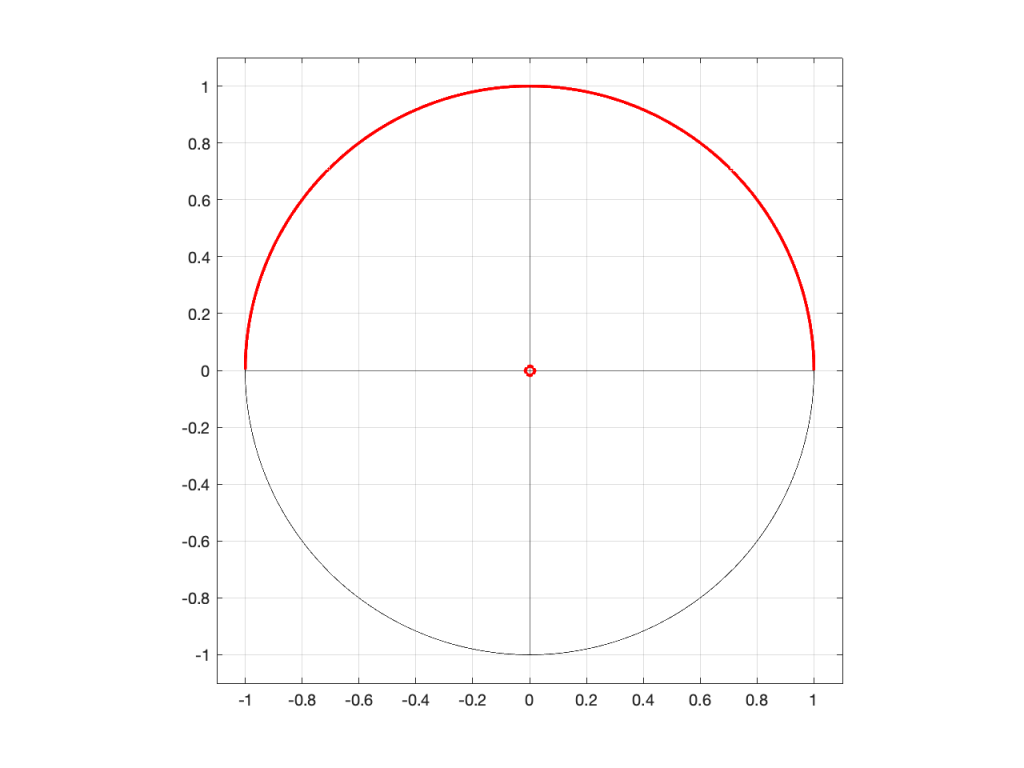Figure 1: A 2D representation of a “zero” (indicated by a red circle) in the middle of the circle that shows the frequency scale (the red line shows the scale going counter-clockwise from 0 Hz on the right to Fs/2 on the left)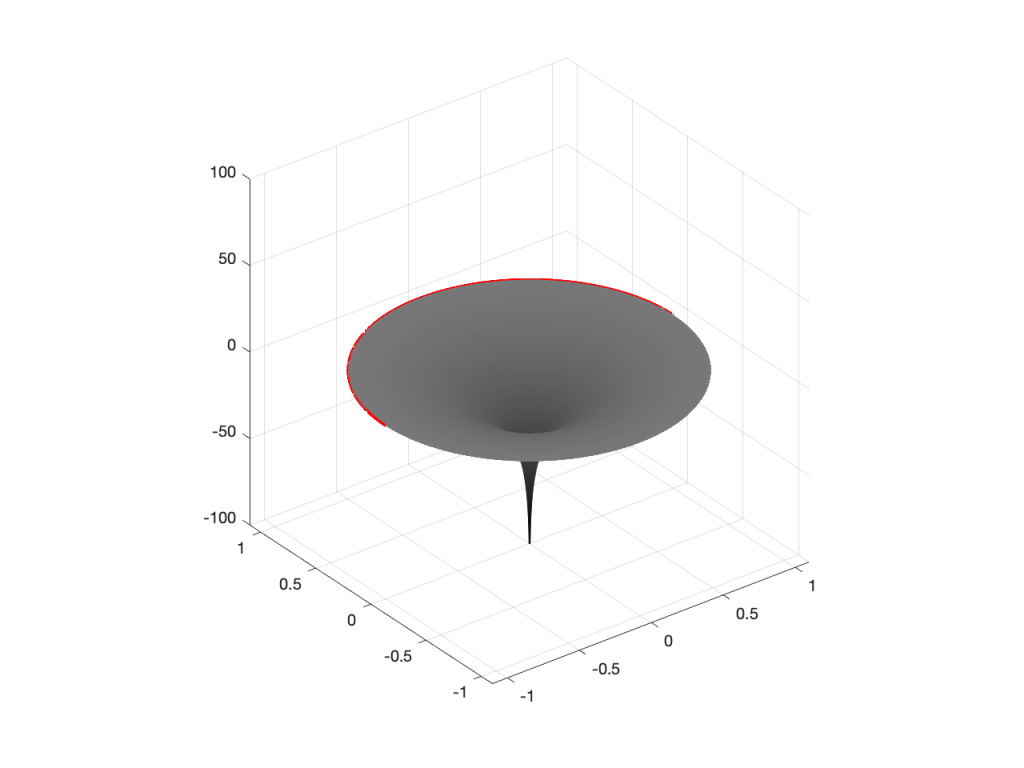Figure 2: A 3D representation of the same information shown in Figure 1.

If I were to put the pole (indicated by a red ‘x’) in the middle of the circle instead, then the result would look like Figures 3 and 4.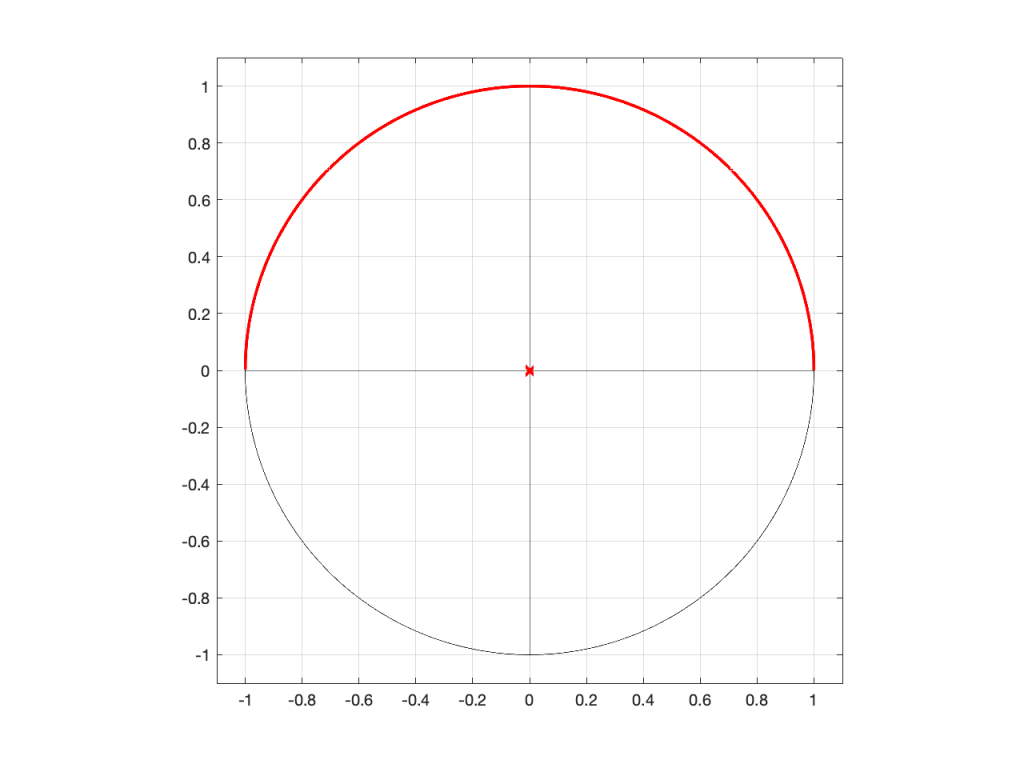Figure 3: A 2D representation of a “pole” (indicated by a red circle) in the middle of the circle that shows the frequency scale (the red line shows the scale going counter-clockwise from 0 Hz on the right to Fs/2 on the left)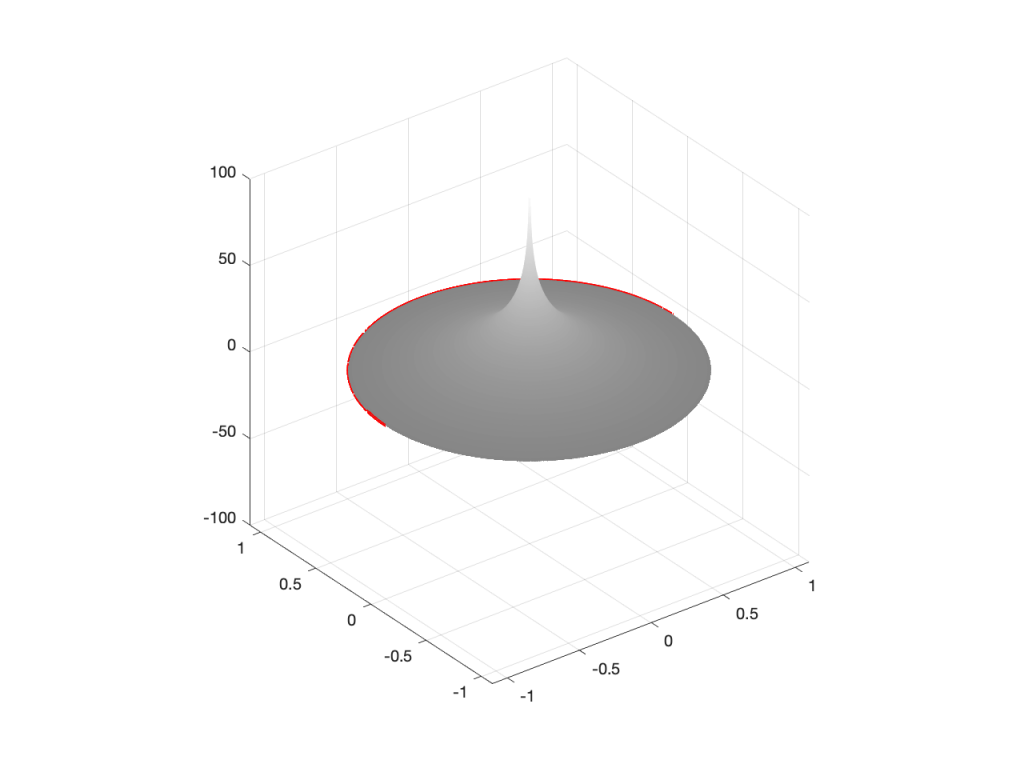Figure 4: A 3D representation of the same information shown in Figure 3.

So far so good… If we were to rotate Figures 2 or 4 and look at the red line that I’ve drawn around the edge, we’d see that it’s flat with a height of 0 (on the vertical scale) all the way around. This is because I’ve carefully placed the zero or the pole at the exact middle of the circle, so it’s pulling equally on all points of the edge of the “tent” or the “funnel”.

However, what would happen if I moved the zero or the pole away from the middle? Some examples of this for a zero moved to the location (-0.75, 0) are shown in Figures 5 to 7, below.

As you can see in Figure 7, when the zero is moved away from the centre of the circle, it pulls downwards on the closer edge (notice how the red line is lower than the black line which has a constant height of 0). However, it also doesn’t pull downwards as much on the opposite side of the circle (notice how the red line is higher than the black line on the left side).

Of course, if I were to do the same thing with a pole, everything would behave symmetrically, as shown in Figures 8 to 10.

We’re almost finished… One more posting to go to wrap up.

# Intuitive Z-plane: Part 3 – More setup

I wrote an intuitive explanation of aliasing in this posting and dug in a little deeper, looking at the side-effects of aliasing with audio signals specifically in this posting.

One of the more important figures in that second posting is repeated below in Figure 1.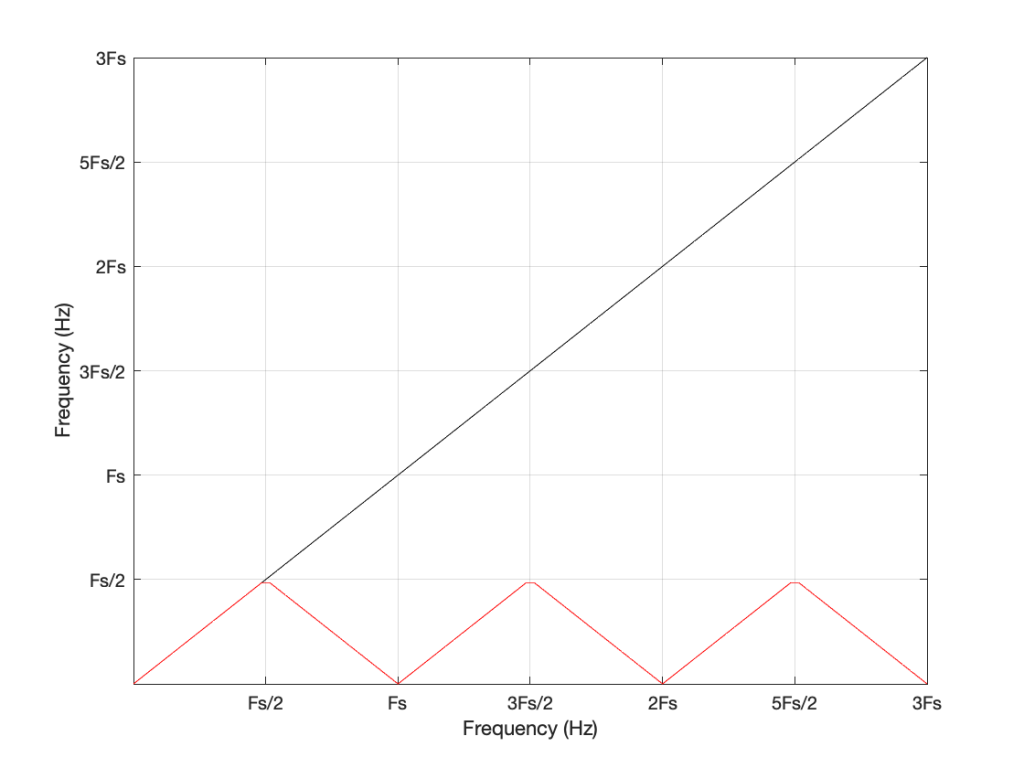Figure 1: The black line shows the intended frequency of a sine wave created in the digital domain, expressed as a fraction of the sampling rate (Fs). The red line shows the actual frequency that comes out of the system as a result – its “alias”.

Let’s say that we wanted to make a sine wave generator in the digital domain. This is pretty easy to do using some rather simple math, as follows:

Output(n) = sin(2 * π * Fc / Fs * n)

where Fc is the frequency of the sine wave in Hz, Fs is the sampling rate in Hz, and n is the time, expressed as a sample number.

There are no restrictions on Fc – so if you wanted to plug in a value that is higher than Fs/2 (the Nyquist frequency) then you’ll get a value. However, if you used this math to try to make a sine wave where Fc > Fs/2, then the output will be different from what you expect. This is what’s shown in Figure 1. The red curve shows the actual frequency of the output (read off the Y-axis) for an intended frequency (on the X-axis).

This problem of the difference between input and output is identical to what would happen if you rotated a wheel by some angle, and then asked someone to measure the rotation. For example, look at Figure 2.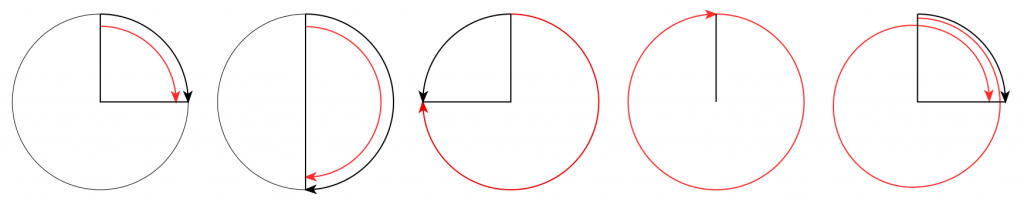Figure 2. The Red arrow shows the actual rotation. The Black arrow shows the assumed rotation.

On the left, it shows a wheel that was rotated clockwise by 90º (indicated by the red arrow). Someone measuring the rotation would say that it was rotated by 90º – a perfect match! If you rotated by 180º (the second example), the person measuring would also get the right answer. However, if you rotated by 270º (the third example, in the middle), the person measuring would (correctly) say that you rotated by 90º counterclockwise. A rotation of 360º gets you back where you started, so it would be measured as 0º. A rotation of 450º (the example on the right) would be measured as a rotation of 90º.

If we were to do this a lot, and plot the results, they’d look like Figure 3.

Now compare Figure 3 to Figure 1. Notice how they’re identical? This is important because it’s a graphic example of exactly the way frequencies “wrap” in a digital audio world. This “wrapping” is the result of the fact that a sinusoidal wave (a signal containing only one frequency) is just a 2-dimensional view of a 3-dimensional rotation (I showed this with photos of a Slinky™ in this posting.

When we normal people look at a magnitude response of a device – let’s say, a low-pass filter, we put it on a nice cartesian plot with the frequency displayed on a straight line on the X-axis and the magnitude displayed on a straight line called the Y-axis. This looks something like Figure 4.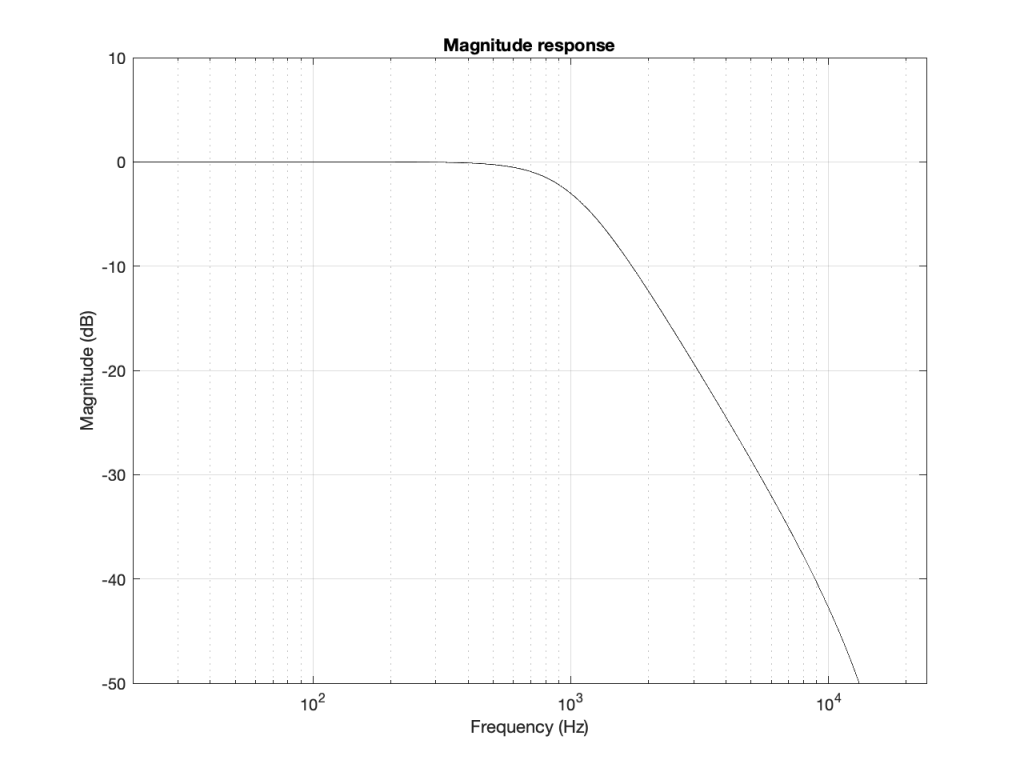Figure 4: A typical, familiar way of viewing a magnitude response of something (in this case, a low-pass filter with Fc=1 kHz).

However, this is only a portion of the truth. The truth extends further than the limits of that plot. I conveniently stopped plotting at Fs/2 (since the filter that I made is running at 48 kHz, this plot goes up to 24 kHz). I also didn’t plot anything below 20 Hz – and I certainly didn’t extend the plot below 0 Hz into the negative frequencies… (“Negative frequencies?” I hear you ask… These are the same as positive frequencies, except that 3-dimensional wheel is rotating in the opposite direction; but since we’re only looking at it on-edge from one location, we can’t tell whether it’s rotating clockwise or counter-clockwise. See this posting if you want to go further.)

Let’s try extending the plot. First, I’ll show Figure 4, but using a linear scale for the frequency instead of a logarithmic scale. This is shown in Figure 5.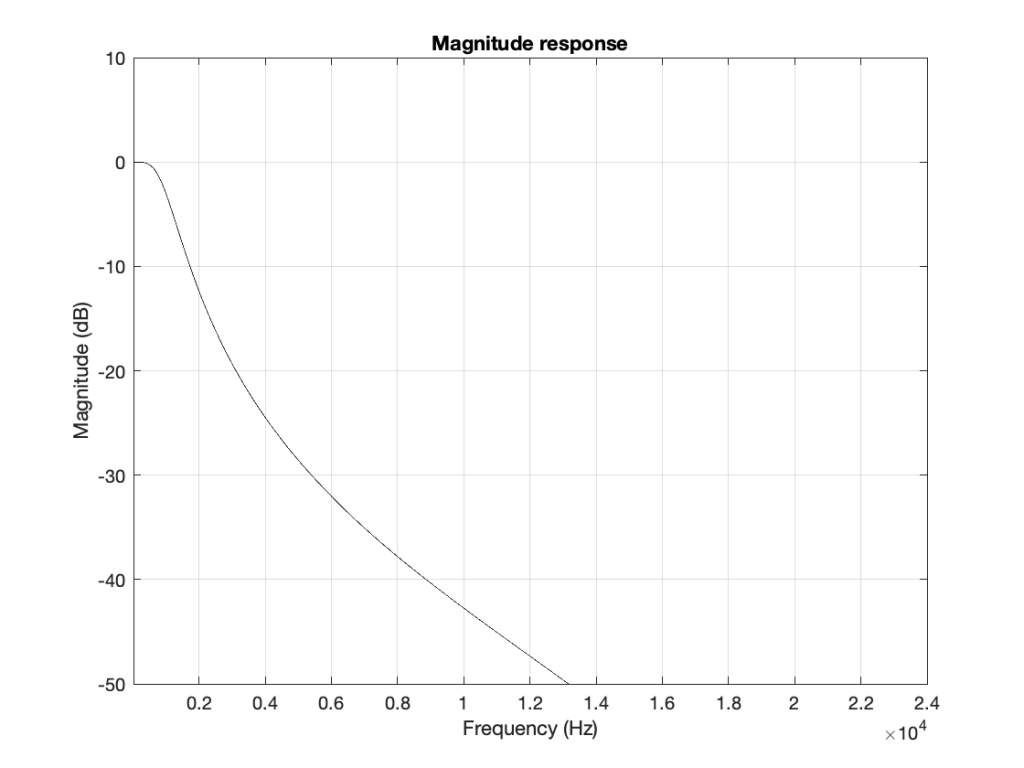Figure 5: The same information shown in Figure 4, but on a linear frequency scale. Notice how 1 kHz is quite low compared to 24 kHz.

If I then were to plot beyond Fs/2, then the magnitude response would be a mirrored version of the one you see in Figure 4. The same would be true if I were to plot below 0 Hz. This is shown in Figure 6.

What does this mean? It means for example that, if I had an LPCM system running at 48 kHz, and I were to digitally generate a sine tone at 48 kHz, then the result would be the same as making a “sine tone” at 0 Hz (or “DC”) because all of the samples would have the same value – neither 0 Hz nor 48 kHz would be a sinusoidal wave in a 48 kHz system. If I then, inside the same system, sent that “48 kHz sine tone” through a low-pass filter with a cutoff frequency of 1 kHz, then it would go through un-impeded (just like a 0 Hz signal would get through a low-pass filter).

## Assembling the pieces

Let’s take the illustration I just showed in Figure 6, and consider it, knowing what I showed in the comparison between Figures 3 and 1.

Although we normal people show each other magnitude responses that look like the one in Figure 4, this is not the way people who make digital signal processing (DSP) software think. They see the frequency axis on a circle that goes from 0 Hz up to Fs/2 (the Nyquist frequency), and then wraps back around to 0 Hz (= Fs). This weird way of viewing the world is shown in Figure 7.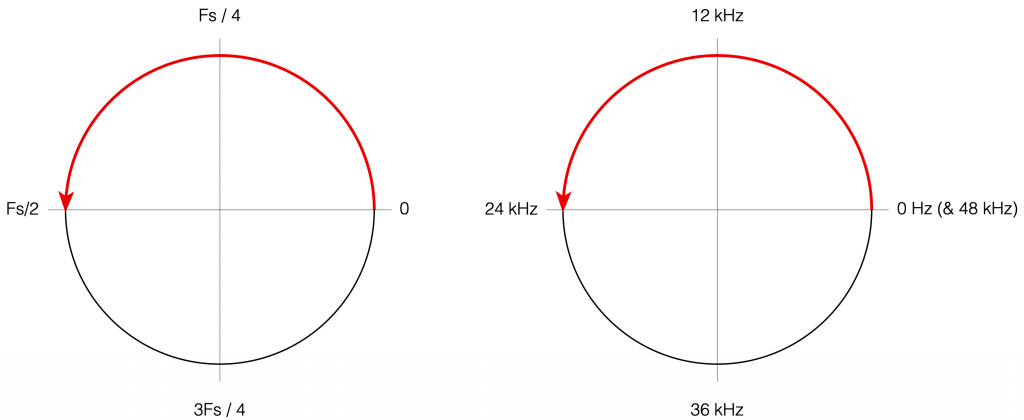Figure 7: DSP engineers think of the frequency axis as the top half of a circle that starts at 0 (Hz) on the right side, and goes (linearly) up to Fs/2 when you get to the opposite side. An example, with a system running at 48 kHz, is shown on the right.

There are some very good reasons why DSP engineers think like this – one of which you already know (the wrapping and aliasing issue). There are some reasons I’m not going to talk about here (but you can read this if you’re interested), and there are some other reasons that I’m headed towards…

However, before we move on to the next chapter in our little saga, it’s best to get really comfortable with the plots in Figure 7. I especially want you to get used to some specific things, in order of importance:

• The frequency scale is circle – it’s not a straight line.
• The scale starts on the right (at the 3 o’clock position) and goes counter-clockwise to the left (the 9 o’clock position).
• The scale is linear, not logarithmic, like you’re used to seeing.
• The maximum frequency is the Nyquist frequency, so it’s defined by the sampling rate.
• Once the point on the circle goes beyond the Nyquist, we’ve started aliasing, and so we’ve entered a symmetrical world that mirrors the half below the Nyquist. (In other words, when we get a little farther, you’ll see that the top and the bottom of that circle are mirror images of each other – as I’ve already hinted in Figure 6 looking at the frequency range from 0 to 48 kHz.)

# Intuitive Z-plane: Part 2 – Peaks and Dips

Most digital filters that are applied to audio signals use a “basic” building block called a “biquadratic filter” or “biquad” which consists of 2 feed-forward delays and 2 feed-back delays, each with its own output gain and a delay time of 1 sample. I’ve already talked a little about biquads in this posting, where I showed a couple of different ways to implement it. One of the standard ways is shown below in Figure 1.

The signal flow that I drew for Figure 1 is a little more modular than the way it’s normally shown, but that’s to keep things separate for the purposes of this discussion.

The two feed-forward delays add to the input signal (via gains b0, b1, and b2) and the result shows up at the red arrow. Remember from Part 1 that this portion of the biquad can only make a magnitude response that has (in an extreme case) infinitely deep, sharp dips, and smooth rounded peaks.

The signal from the red arrow onwards goes into the feed-back portion of the filter with two feed-back delays adding through gains -a1 and -a2. Again, remember from Part 1 that this portion of the biquad can make a magnitude response that has infinitely deep, sharp peaks, and smooth rounded dips.

Let’s say that we wanted to make a simple filter – let’s make it a low pass filter – using this biquad. How do we do it?

The simplest way is to cheat and go straight to the answer.

Cheating Option 1: You go to this page at www.earlevel.com and put in the parameters you’re interested in (Filter Type, sampling rate, Fc, Q, etc…) and copy-and-paste the resulting five gains (we’ll call them “coefficients” from now on).

Cheating Option 2: We search on the Interweb for the words “RBJ Audio Cookbook” and then spend some time copying, pasting, and porting the equations that Robert Bristow-Johnson bestowed upon us many years ago* into your processor. You then say “I want a low pass filter at 1000 Hz with a Q of 0.5, please” and the equations spit out the five coefficients that you seek.

However, if you cheat, you’ll never really get a grasp of how those coefficients work and what they’re really doing – and that’s where we’re headed in this little series of articles. So, you might decide to go through this series, and then cheat afterwards (that’s what I would recommend…)

Now, before you go any further, I’ll warn you – the whole purpose of this series is to give you an intuitive understanding. This means that there are things I’m going to (intentionally) skip over, merely mention in passing, or omit completely. So, if you already know what I’m talking about, there’s no point in reading what I’m writing – and there’s certainly no need to email me to remind me that I didn’t mention some aspect of this that you think is important, but I’ve decided is not. If you feel strongly about this, write your own blog.

## P.S.

* Thanks, Robert!